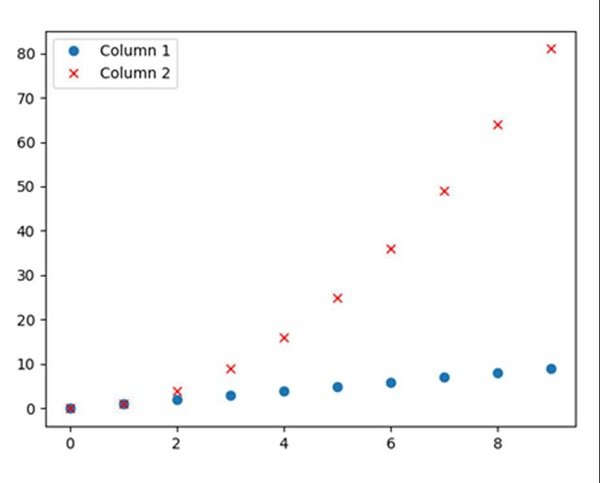# How to plot two columns of a Pandas data frame using points?

First, we can initialize the dictionary with col1 and col2, convert it into a data frame. After that, we can plot this data with ‘o’ and ‘rx’ style.

## Steps

• Create a dictionary with Column 1 and Column 2 as the keys and Values are like i and i*i, where i is from 0 to 10, respectively.

• Create a data frame using pd.DataFrame(d); d created in step 1.

• Plot the data frame with ‘o’ and ‘rx’ style.

• To show the plot, use plt.show().

## Example

import pandas as pd
from matplotlib import pyplot as plt

d = {'Column 1': [i for i in range(10)], 'Column 2': [i*i for i in range(10)]}

df = pd.DataFrame(d)

df.plot(style=['o', 'rx'])

plt.show()

## Output